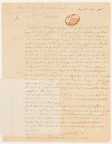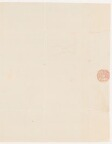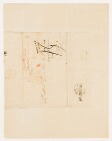Crédit photographique : Bibliothèque de l’Institut de France

ET3733 réponses

Sélectionner par

• Année
Sans date
1760
1770
1780
1790
→ Aucune sélection

• Marques postales
Non
Oui
→ Aucune sélection

• Type de contenu
Description du papier
Image du filigrane
Transcription
Transcription & papier
→ Aucune sélection

Notice
Identification et lieu de conservation
IDC544
TitrePatrick BROWNE à CONDORCET - 11 août 1789 (Paris, Archives de l’Académie des sciences, pochette de la séance du 12 août 1789)
Nature du documentOriginal
Lieu de conservationParis, Archives de l’Académie des sciences
Cotepochette de la séance du 12 août 1789
Intervention(s)
Expéditeur(s) et destinataire(s)
Instrument d’écriturePlume trempée dans l’encre noire
Dates
Date indiquée par le scripteurAugust. 11. 1789
Datation11 août 1789
Date de réception[inconnue]
Note(s) dates

Cette lettre a sans doute été associée a posteriori à la Pochette de séance du 12 août 1789 car elle date de la veille même. [EVZ] Note allographe au crayon à ce sujet - mais qui semble indiquer 18 ?

Lieux
Lieu d'écriture indiqué par le scripteurHiberno-Tuamensis in Hibernia

Lieu d'écriture rétabli ou normaliséTuam
Lieu d'écriture indexé
Lieu de destination indiqué par le scripteur[néant]
Lieu de destination rétabli ou normaliséParis, Académie des sciences
Lieu de destination indexé

A Monsieur Monsieur Le Secrétaire de l'Académie Royal [sic] des Sciences Paris

Note(s) lieu(x)

Hibernia désigne l'Irlande, en latin.

Marque(s) postale(s)
Marque(s) postale(s)Oui
Pliage d’expéditionPli cacheté
Tarif postal[international ; marques multiples]
Marque du bureau d’expéditionTUAM
Bureau d'expédition indexé
Note(s) marque(s) postale(s)

Marques manuscrites :

• Post [?] Irish -- 4
• British -- 6

Tampon à l'arrière du pli :

• un cercle "U 89" et 2[?]

Marque manuscrite : [95 ?]

Tampon circulaire très effacé ou transfert.

Papier et cachet
Description sommaire du papier

Bifeuillet in-4°, vergé écru, filigrané

Textes

Ouvrir dans une nouvelle page

Note sur la transcription : Browne écrit souvent les mots that, the et which sous une forme aujourd’hui archaïque, soit respectivement yt, ye, et w.h. Afin de faciliter la lecture, nous avons rétabli la forme moderne.

## Transcription

[1 r] August. 11 1789.

Sir,

Inclosed I send the Diagram for my method of squaring the Circle, which is compleat, without any fraction, and every part of the Demonstration geometrical which is underneath. To be laid before the Royal Academy.

|Preparation.| The Circle is inscribed in a square equall to the Diamiter of the Circle, and within the Circle is another square, whose sides are equall to, or rather, the whole 4 Chords, and by perpendiculars on these |continued.| The circumscribed square is divided into 16 equall triangles ($m$). The inscribed square is made up of eight of the same triangles, & the Circle contains these .8. triangles ($m$) and four segments $\mathrm{SS}$. Now we are to prove that the four segments are equall to 4 triangles & then the Area of the Circle will be equal to 12 triangles. And of course equal to $\frac{3}{4}$ers of the Area of the square circumscribed to the Circle; or equall to the whole +$\frac{1}{2}$ of the inscribed square: I must premise that a considerable part of the foundation of this Demonstration depends on the proposition of Euclid which proves that the square of the Hypothenuse is equall to the squares of the two other sides, and consequently that the surfaces of regular & similar figures are as the squares of their Homologous Sides. This posed.

Raise the half or oblong square $\mathrm{DLMQ}$, by continuing the Chords $\mathrm{HD.GE}$ and drawing the line $\mathrm{LM}$ at the top, and you have 2 small squares, each equall to and divided into 2. triangles $m$.

On the Chord $\mathrm{DQ}$ (one of the sides of the right angled triangle $\mathrm{DGQ}$) draw the semicircle $\mathrm{DKQ}$, and you will have the semicircle equall to the Quadrant (proposition). But the semicircle and the quadrant have the segment $\mathrm{SS}$ in common, therfore taking away the segmt $\mathrm{SS}$, the Lunula as, as is equal to the triangle 3 = triangle 4 = one of the small squares and from thence the semi lunula as = one triangle, $m$, and $S$ will be half $\mathrm{SS}$.

Now $\mathrm{Sas}$ = triangle + $s$ |or $S$| take away $a$, remains $S$ = $s$ & $\mathrm{asB}$ = triangle + $a$ or $b$. Take away $s$ & remains $a=b$.

[1 v] Now you have & . . And by taking away as the Lunula, remains $S=b$ & both together equall to half the little square or one triangle. Then you have .

Yet as there is so little similarity between $a$ & $S$ I thought proper to give another demonstrative proof of their equality. Look therefore to the next small square wh is divided in the same manner, and further divided by a cross Diagonal into 4 equall triangles $\mathrm{xxxx}$, each equal to half a triangle, $m$; and this Diagonal divides also $S$, into $N+o$, and $a$, into $L+z$. Then say

and . Take away .$o$. from both sides and you have . Take away $z$ from both of the $L$ out and you have $L=N$. Now $L+o$ is a triangle $x$ therefore $N=L+o=x$. |But & $L+o$ are equal| and by taking away $L$ & $N$ you have $z=o$.

I have shown that |= $x$|, and $N+o=x=S$. Therefore , q. e. d.

Now $\mathrm{SS}$ |the segment| = $\mathrm{Sa}$ the triangle, $m$, therefore the 4 segments equal four triangles $m$. And therefore the Circle equalls 12 triangles or $\frac{3}{4}$ers of the Area of the square circumscribed to the Circle or once & a half the prescribed square, q.e.d.

Now suppose a Circle of 10 Diameter, the Area of one triangle will be, $6.25×12=75$ and $\frac{3}{4}$ of $10×10=100$ . év $75$.

And the square of the rad. multiplied by 3 is $25×3=75$.

And the solidity of the sphere = $\frac{1}{2}$ the Cube, having lost $\frac{1}{4}$ in the Angles & $\frac{1}{4}$ in the sloping or = $\frac{2}{3}$ of the Cylinder layed on the Area of the Circle and $\frac{1}{3}$ of the Cylinder = $\frac{1}{4}$ of the Cube.

I remain Sir Your Very obedt & humble Servt

Patrick Browne MD.

Hiberno-Tuamensis in Hibernia

[2 r vierge] [2 v] [Adresse et marques postales]
1234Responsable du projet : Nicolas Rieucau, Laboratoire d'économie dionysien (EA 3391) / Université Paris VIII.
Projet financé par l'Agence Nationale de la Recherche (ANR)
Hébergement du site : TGIR Huma-Num

© 2016 - 2021 Laboratoire d’économie dionysien

Dernière mise à jour: dimanche 1 octobre 2023 (19:25) +
Rendu de la page en 0.033s